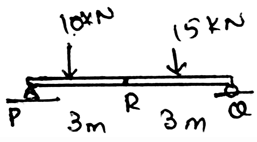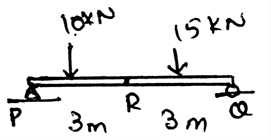Courses

# Test: Shear And Moment Equations And Diagrams

## 15 Questions MCQ Test Engineering Mechanics | Test: Shear And Moment Equations And Diagrams

Description
This mock test of Test: Shear And Moment Equations And Diagrams for Mechanical Engineering helps you for every Mechanical Engineering entrance exam. This contains 15 Multiple Choice Questions for Mechanical Engineering Test: Shear And Moment Equations And Diagrams (mcq) to study with solutions a complete question bank. The solved questions answers in this Test: Shear And Moment Equations And Diagrams quiz give you a good mix of easy questions and tough questions. Mechanical Engineering students definitely take this Test: Shear And Moment Equations And Diagrams exercise for a better result in the exam. You can find other Test: Shear And Moment Equations And Diagrams extra questions, long questions & short questions for Mechanical Engineering on EduRev as well by searching above.
QUESTION: 1

### ___________ are the structures that are being made to support the loadings applied perpendicular to the axis of that structures.

Solution:

The beams are the structures which are being made so as to support the loadings which are perpendicular to the axis of that structure. This means that the beams can support that weight or that types of weight which are applying the load perpendicular to the axis of the beam.

QUESTION: 2

### Beams are long and ________ structures that are being made to support the loadings.

Solution:

Beams are long and straight structures that are being made to support the loadings. They are being made so as to support the loadings which are perpendicular to the axis of that structure. This means that the beams can support that weight or that types of weight which are applying the load perpendicular to the axis of the beam.

QUESTION: 3

### Beams have a _________ cross sectional area.

Solution:

Beams have a uniform cross sectional area. This is because beams are the structures which are being made so as to support the loadings which are perpendicular to the axis of that structure. And to support properly the area must be uniform.

QUESTION: 4

Simply supported beams are pinned at both the ends.

Solution:

The simply supported beams are supported only at one side by the pin. This means the other side is having the roller. The beams are such designed because they are the structures which are being made so as to support the loadings which are perpendicular to the axis of that structure. Thus not pinned both sides.

QUESTION: 5

The other side of the simply supported beam is having pin support, what is the support this side?

Solution:

The simply supported beams are supported only at one side the pin. This means the other side is having the roller. The beams are such designed because they are the structures which are being made so as to support the loadings which are perpendicular to the axis of that structure. Thus not pinned both sides.

QUESTION: 6

The cantilever beam is having pin supports both sides of it.

Solution:

The one end of the cantilever beam is fixed and the other one is having its end as free. This is the other type of the beam which is being designed to support the loadings which are perpendicular to the support. Thus the cantilever is free from one end and fixed at another.

QUESTION: 7

The cantilever is having one side of it as fixed while the other one is _________

Solution:

The one end of the cantilever beam is fixed and the other one is having its end as free. This is the other type of the beam which is being designed to support the loadings which are perpendicular to the support. Thus the cantilever is free from one end and fixed at another.

QUESTION: 8

The design of the beam requires the knowledge of the variation of the ____________

Solution:

The design of the beam requires the knowledge of the variation of the internal shear force. Because beams are the structures which are being made so as to support the loadings which are perpendicular to the axis of that structure. And to support properly the area must be uniform.

QUESTION: 9

The design of the beam requires the knowledge of the variation of the ____________

Solution:

The design of the beam requires the knowledge of the variation of the bending moment. Because beams are the structures which are being made so as to support the loadings which are perpendicular to the axis of that structure. And to support properly the variation of the bending moments must be known for each of the section.

QUESTION: 10

The bending moment and the shearing stress can be obtained by which method?

Solution:

The design of the beam requires the knowledge of the variation of the bending moment. Because beams are the structures which are being made so as to support the loadings which are perpendicular to the axis of that structure. Thus to know these quantities we apply the method of sections so as to determine these values easily.

QUESTION: 11

We can make the graph of shear force acting on the beam. But not for the bending moment.

Solution:

Both of them can be plotted on the graph as the main things are the distance variation of the force, which can be made by simple calculation. The main motto of the calculations are to design the graph and know where the maximum force and the moment are. Thus the graph of both the quantities are possible.

QUESTION: 12

We apply the equations of ________ to determine various forces acting on the beams.

Solution:

The force developed by a support doesn’t allow the translation of its attached member. This is the basic condition for the equilibrium of the forces in any dimension. And many other are applied at the points where the forces are to be determined. Thus equilibrium equations are being applied at the points where the main forces are to be determined.

QUESTION: 13

Which of the following is true for a beam having various forces acting over it?

Solution:

We can take such assumptions. We are free to do so. We just need to specify if clockwise is positive then the counter clockwise is to be negative. Also such assumption are very useful in 2D calculation of the moments caused by various forces on the body.

QUESTION: 14

Determine the shear force generated.Solution:

The loading acting upward is considered to be positive. This is done as to decrease the time used for the calculations. If the method of sections is being applied at the complex sections for the determination of the unknown forces, much time is required for it. Thus the main motto is to make the calculations easy and make the less use of time.

QUESTION: 15

Determine the moment generated at R.Solution:

The loading acting upward is considered to be positive. This is done as to decrease the time used for the calculations. If the method of sections is being applied at the complex sections for the determination of the unknown forces, much time is required for it. Thus the main motto is to make the calculations easy and make the less use of time.